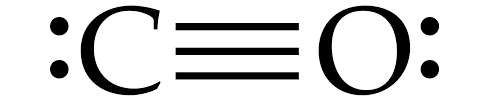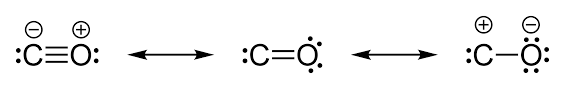# Carbon monoxide has a ………. bond between C and O.(A) Triple(B) Double (C) Single(D) Ionic

Last updated date: 29th Jan 2023
Total views: 261.6k
Views today: 6.55kVerified
261.6k+ views
Hint: There are two covalent bonds and one coordinate bond present in Carbon monoxide molecules. The bond order of Carbon monoxide is three. So, the bond between C and O is strong enough.

In CO, there is a triple bond between C and O.
Out of these three bonds, two are formed by sharing of electrons (covalent bond) between C and O. And one bond is a coordination bond, in which the Oxygen donated its lone pair of electrons to the Carbon atom.
Structure of Carbon monoxide is given below:Carbon monoxide is linear in structure , with a bond angle of ${ 180 }^{ 0 }$ in between C and O atoms.
As we know that one of the bonds in CO is a coordination bond, which is formed by the donation of lone pairs of electrons from Oxygen to Carbon atoms.
We should know one more thing here that, since the electrons are being donated from Oxygen atom to Carbon atom, therefore, in actual structure of Carbon monoxide, there exists a partial positive charge on Oxygen atom and Partial negative charge on Carbon atom as shown below:From this, we can get to know that there is a triple bond in between C and O in CO molecules.
Therefore, Option (A) triple bond is the correct answer.

Additional Information: Carbon monoxide is also a well known ${ \pi }$ -acid ligand / ${ \pi }$ acceptor ligand.
A ${ \pi }$ acid ligand is a ligand that can form an additional double bond by accepting a lone pair of electrons from the metal, along with the normal ${ \sigma }$ bonds .

Note: One can also explain this by the bond number of CO molecule, which is determined by the equation $\frac { 1 }{ 2 } \left( { N }_{ b }-{ N }_{ a } \right)$ , where ${ N }_{ b }$ is the number of electrons in the bonding orbital and ${ N }_{ a }$ is the number of electrons in antibonding orbitals. In the case of CO, this value comes out to be 3 which implies that it has a triple bond.